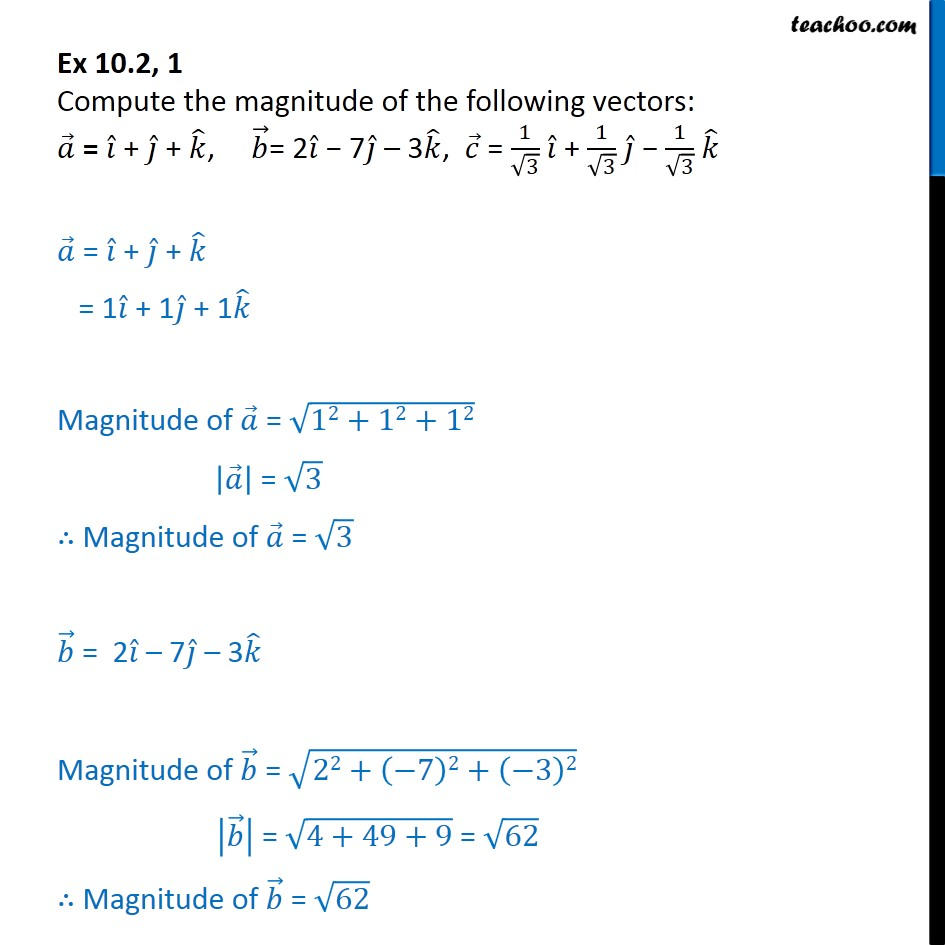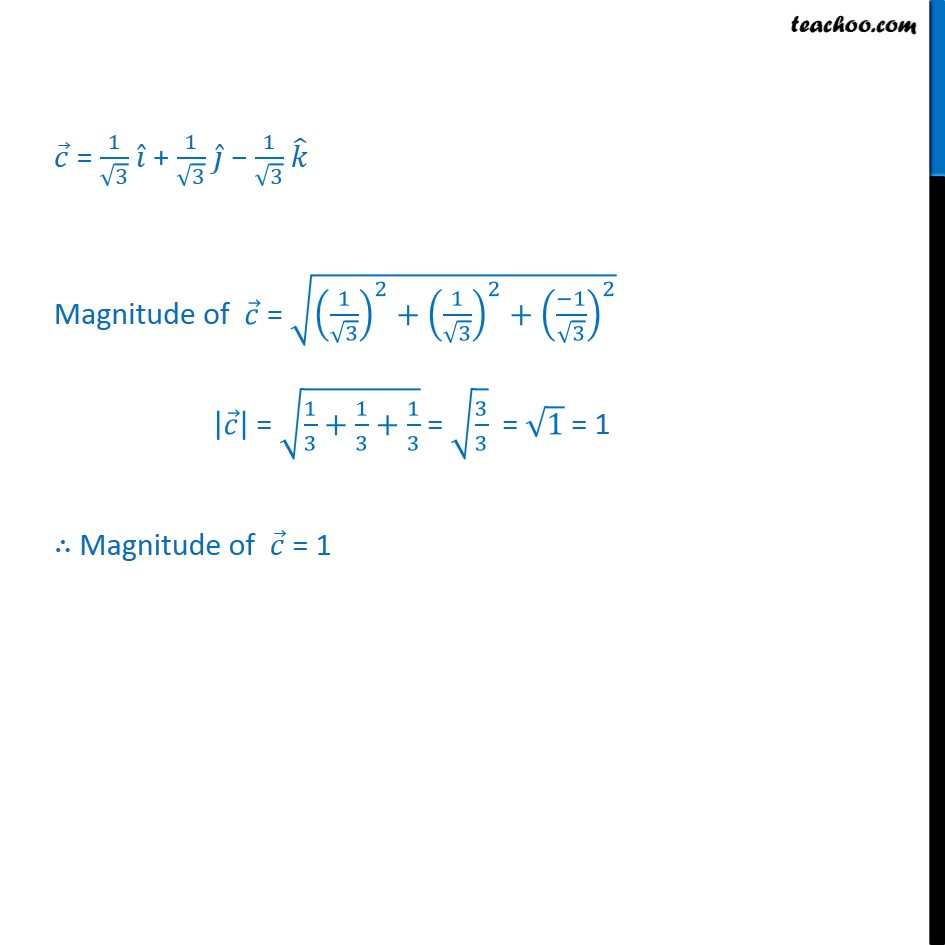Subscribe to our Youtube Channel - https://you.tube/teachoo

1. Chapter 10 Class 12 Vector Algebra
2. Serial order wise
3. Ex 10.2

Transcript

Ex 10.2, 1 Compute the magnitude of the following vectors: = + + , = 2 7 3 , = 1 3 + 1 3 1 3 = + + = 1 + 1 + 1 Magnitude of = 12+12+12 = 3 Magnitude of = 3 = 2 7 3 Magnitude of = 22+ 7 2+ 3 2 = 4+49+9 = 62 Magnitude of = 62 = 1 3 + 1 3 1 3 Magnitude of = 1 3 2 + 1 3 2 + 1 3 2 = 1 3 + 1 3 + 1 3 = 3 3 = 1 = 1 Magnitude of = 1

Ex 10.2

About the AuthorDavneet Singh
Davneet Singh is a graduate from Indian Institute of Technology, Kanpur. He has been teaching from the past 9 years. He provides courses for Maths and Science at Teachoo.